# Hinton神经网络公开课编程练习1 The perceptron learning algorithm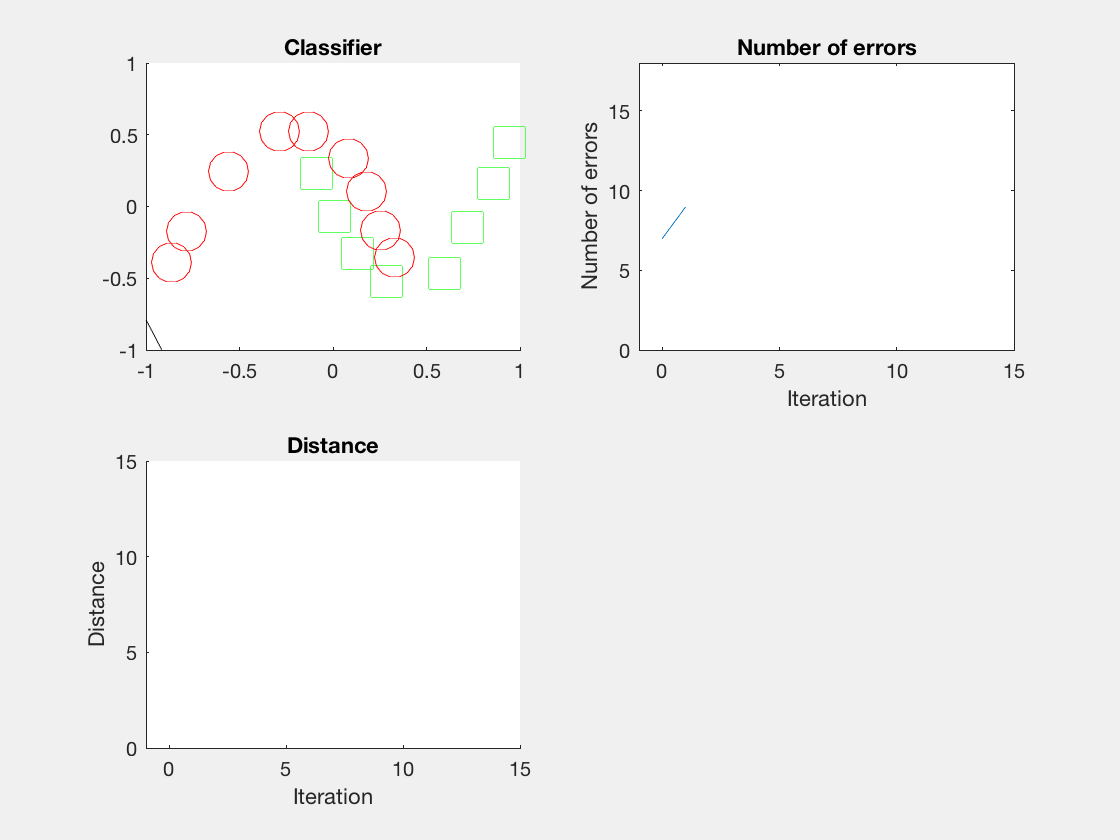这门课的编程练习很简单，大部分代码都给出了，只需要修改或添加一小部分。官方语言为matlab，但我更喜欢Python（讨厌jupyter)。但matlab保存动画更方便，所以决定双语字幕，左右开弓。

## 算法主框架

```def learn_perceptron(neg_examples_nobias, pos_examples_nobias, w_init, w_gen_feas,
pause=False):
"""Learns the weights of a perceptron for a 2-dimensional dataset and plots
the perceptron at each iteration where an iteration is defined as one
full pass through the data. If a generously feasible weight vector
is provided then the visualization will also show the distance
of the learned weight vectors to the generously feasible weight vector.

Args:
neg_examples_nobias (numpy.array) : The num_neg_examples x 2 matrix for the examples with target 0.
num_neg_examples is the number of examples for the negative class.
pos_examples_nobias (numpy.array) : The num_pos_examples x 2 matrix for the examples with target 1.
num_pos_examples is the number of examples for the positive class.
w_init (numpy.array)              : A 3-dimensional initial weight vector. The last element is the bias.
w_gen_feas (numpy.array)          : A generously feasible weight vector.
pause (bool)                      : Pause between iterations.
Returns:
numpy.array : The learned weight vector.
"""
num_err_history = []
w_dist_history = []

# add column vector of ones for bias term
neg_examples = np.hstack((neg_examples_nobias, np.ones((len(neg_examples_nobias), 1))))
pos_examples = np.hstack((pos_examples_nobias, np.ones((len(pos_examples_nobias), 1))))

if np.size(w_init):
w = np.random.rand(3, 1)
else:
w = w_init

if not np.size(w_gen_feas):
w_gen_feas = []

# Find the data points that the perceptron has incorrectly classified
# and record the number of errors it makes.
iter_ = 0
mistakes0, mistakes1 = eval_perceptron(neg_examples, pos_examples, w)
num_errs = len(mistakes0) + len(mistakes1)
num_err_history.append(num_errs)
print "Number of erros in iteration {0}:\t{1}".format(iter_, num_errs)
print "Weights:", w
plot_perceptron(neg_examples, pos_examples, mistakes0, mistakes1, num_err_history,
w, w_dist_history)

# If a generously feasible weight vector exists, record the distance
# to it from the initial weight vector
if len(w_gen_feas) != 0:
w_dist_history.append(np.linalg.norm(w - w_gen_feas))

while num_errs > 0:
iter_ = iter_ + 1

# Update weights of perceptron
w = update_weights(neg_examples, pos_examples, w)

# If a generously feasible weight vetor exists, record the distance
# to it from the current weight vector
if len(w_gen_feas) != 0:
w_dist_history.append(np.linalg.norm(w - w_gen_feas))

# Find the data points that the perceptron has incorrectly classified
# and record the number of errors it makes.
mistakes0, mistakes1 = eval_perceptron(neg_examples, pos_examples, w)
num_errs = len(mistakes0) + len(mistakes1)
num_err_history.append(num_errs)
print "Number of erros in iteration {0}:\t{1}".format(iter_, num_errs)
print "Weights:", w

plot_perceptron(neg_examples, pos_examples, mistakes0, mistakes1, num_err_history,
w, w_dist_history)
if pause:
while True:
try:
ans = input("Continue?")
if ans == 1 or ans == 'y':
break
if ans == 0 or ans == 'n':
return w
except (ValueError, NameError):
print("Sorry, I didn't understand that.")
continue
return w```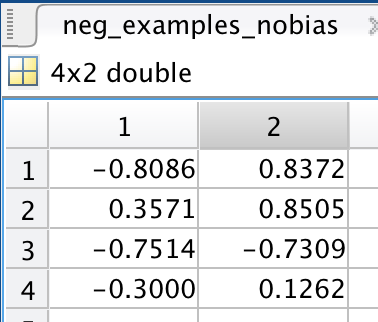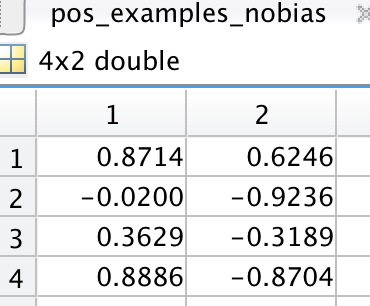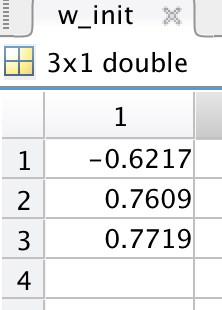## 评估性能

```def eval_perceptron(neg_examples, pos_examples, w):
"""Evaluates the perceptron using a given weight vector. Here, evaluation
refers to finding the data points that the perceptron incorrectly classifies.

Args:
neg_examples (numpy.array) : The num_neg_examples x 3 matrix for the examples with target 0.
num_neg_examples is the number of examples for the negative class.
pos_examples (numpy.array) : The num_pos_examples x 3 matrix for the examples with target 1.
num_pos_examples is the number of examples for the positive class.
w (numpy.array)            : A 3-dimensional weight vector, the last element is the bias.
Returns:
(tuple) :
mistakes0 : A vector containing the indices of the negative examples that have been
incorrectly classified as positive.
mistakes1 : A vector containing the indices of the positive examples that have been
incorrectly classified as negative.
"""
mistakes0 = [i for i, sample in enumerate(neg_examples) if np.dot(sample, w) >= 0]
mistakes1 = [i for i, sample in enumerate(pos_examples) if np.dot(sample, w) < 0]
return mistakes0, mistakes1```

## 权值更新

```def update_weights(neg_examples, pos_examples, w_current):
"""Updates the weights of the perceptron for incorrectly classified points
using the perceptron update algorithm. This function makes one sweep over
the dataset.

Args:
neg_examples (numpy.array) : The num_neg_examples x 3 matrix for the examples with target 0.
num_neg_examples is the number of examples for the negative class.
pos_examples (numpy.array) : The num_pos_examples x 3 matrix for the examples with target 1.
num_pos_examples is the number of examples for the positive class.
w_current (numpy.array)    : A 3-dimensional weight vector, the last element is the bias.
Returns:
(numpy.array) : The weight vector after one pass through the dataset using the perceptron
learning rule.
"""
w = w_current
for sample in neg_examples:
assert len(np.shape(sample)) == 1 and np.shape(w) == 1
activation = np.dot(sample, w)
if activation >= 0:
w += np.column_stack(sample).T * (0.0 - activation)
for sample in pos_examples:
assert len(np.shape(sample)) == 1 and np.shape(w) == 1
activation = np.dot(sample, w)
if activation < 0:
w += np.column_stack(sample).T * (1.0 - activation)
return w```

## 可视化

```def plot_perceptron(neg_examples, pos_examples, mistakes0, mistakes1,
num_err_history, w, w_dist_history):
"""The top-left plot shows the dataset and the classification boundary given by
the weights of the perceptron. The negative examples are shown as circles
while the positive examples are shown as squares. If an example is colored
green then it means that the example has been correctly classified by the
provided weights. If it is colored red then it has been incorrectly classified.
The top-right plot shows the number of mistakes the perceptron algorithm has
made in each iteration so far.

The bottom-left plot shows the distance to some generously feasible weight
vector if one has been provided (note, there can be an infinite number of these).
Points that the classifier has made a mistake on are shown in red,
while points that are correctly classified are shown in green.

The goal is for all of the points to be green (if it is possible to do so).

Args:
neg_examples    : The num_neg_examples x 3 matrix for the examples with target 0.
num_neg_examples is the number of examples for the negative class.
pos_examples    : The num_pos_examples x 3 matrix for the examples with target 1.
num_pos_examples is the number of examples for the positive class.
mistakes0       : A vector containing the indices of the datapoints from class 0 incorrectly
classified by the perceptron. This is a subset of neg_examples.
mistakes1       : A vector containing the indices of the datapoints from class 1 incorrectly
classified by the perceptron. This is a subset of pos_examples.
num_err_history : A vector containing the number of mistakes for each
iteration of learning so far.
w               : A 3-dimensional vector corresponding to the current weights of the
perceptron. The last element is the bias.
w_dist_history  : A vector containing the L2-distance to a generously
feasible weight vector for each iteration of learning so far.
Empty if one has not been provided.
"""
f = plt.figure(1)

neg_correct_ind = np.setdiff1d(range(len(neg_examples)), mistakes0)
pos_correct_ind = np.setdiff1d(range(len(pos_examples)), mistakes1)
assert all(m_idx not in set(neg_correct_ind) for m_idx in mistakes0) and \
all(m_idx not in set(pos_correct_ind) for m_idx in mistakes1)

plt.subplot(2, 2, 1)
plt.hold(True)
if np.size(neg_examples):
plt.plot(neg_examples[neg_correct_ind][:, 0], neg_examples[neg_correct_ind][:, 1], 'og', markersize=10)
if np.size(pos_examples):
plt.plot(pos_examples[pos_correct_ind][:, 0], pos_examples[pos_correct_ind][:, 1], 'sg', markersize=10)

if len(mistakes0):
plt.plot(neg_examples[mistakes0][:, 0], neg_examples[mistakes0][:, 1], 'or', markersize=10)
if len(mistakes1):
plt.plot(pos_examples[mistakes1][:, 0], pos_examples[mistakes1][:, 1], 'sr', markersize=10)

plt.title('Perceptron Classifier')
# In order to plot the decision line, we just need to get two points.
plt.plot([-5, 5], [(-w[-1] + 5 * w) / w, (-w[-1] - 5 * w) / w], 'k')
plt.xlim([-1, 4])
plt.ylim([-2, 2])
plt.hold(False)

plt.subplot(2, 2, 2)
plt.plot(range(len(num_err_history)), num_err_history)
plt.xlim([-1, max(15, len(num_err_history))])
plt.ylim([0, len(neg_examples) + len(pos_examples) + 1])
plt.title('Number of errors')
plt.xlabel('Iteration')
plt.ylabel('Number of errors')

plt.subplot(2, 2, 3)
plt.plot(range(len(w_dist_history)), w_dist_history)
plt.xlim([-1, max(15, len(num_err_history))])
plt.ylim([0, 15])
plt.title('Distance')
plt.xlabel('Iteration')
plt.ylabel('Distance')
plt.show()```

## 调用方法

```import matplotlib.pylab as pylab

pylab.rcParams['figure.figsize'] = 12, 8

import scipy.io
import os
import matplotlib.pyplot as plt

data_path = os.path.join(os.getcwd(), 'data/')
files = ['dataset%d' % i for i in range(1, 5)]

dataset_file = os.path.join(data_path, files)

w = learn_perceptron(data['neg_examples_nobias'],
data['pos_examples_nobias'],
data['w_init'],
data['w_gen_feas'])```

### 结果

Python是这个效果：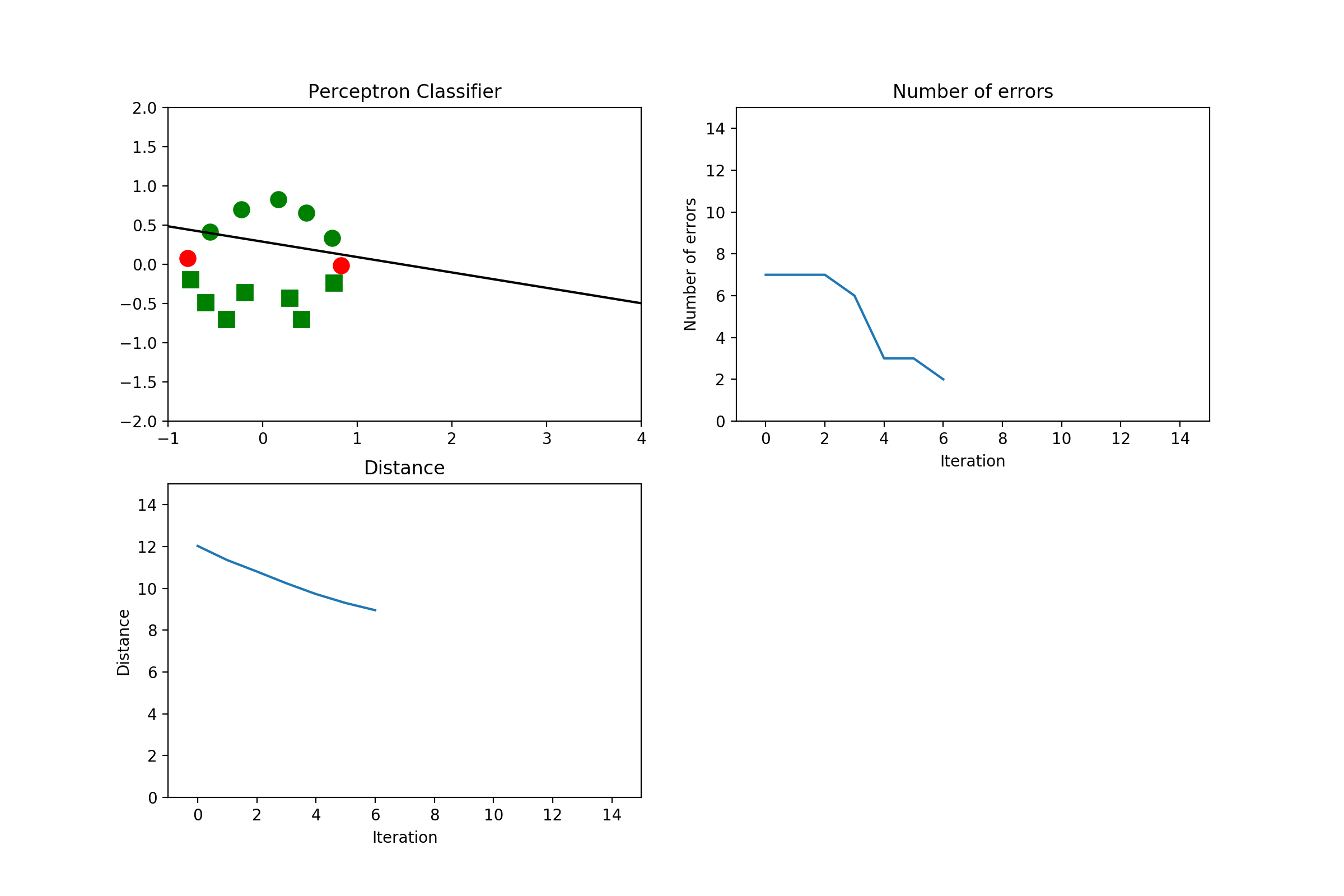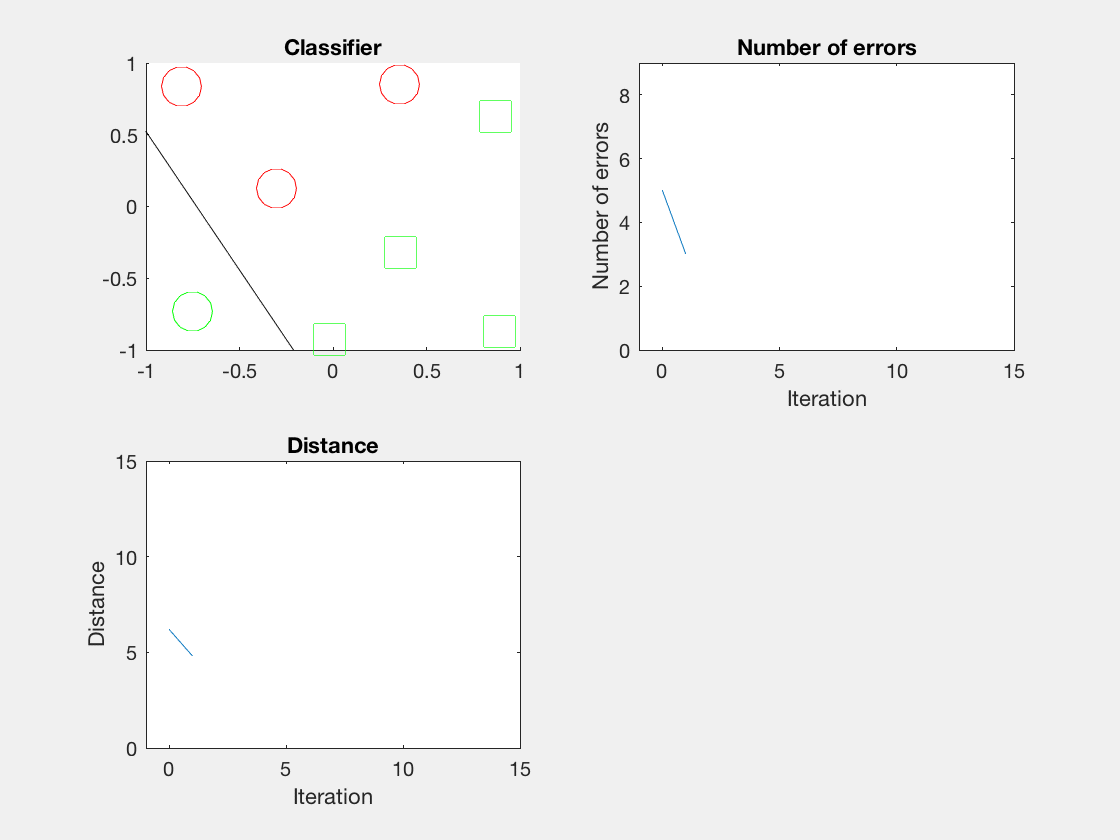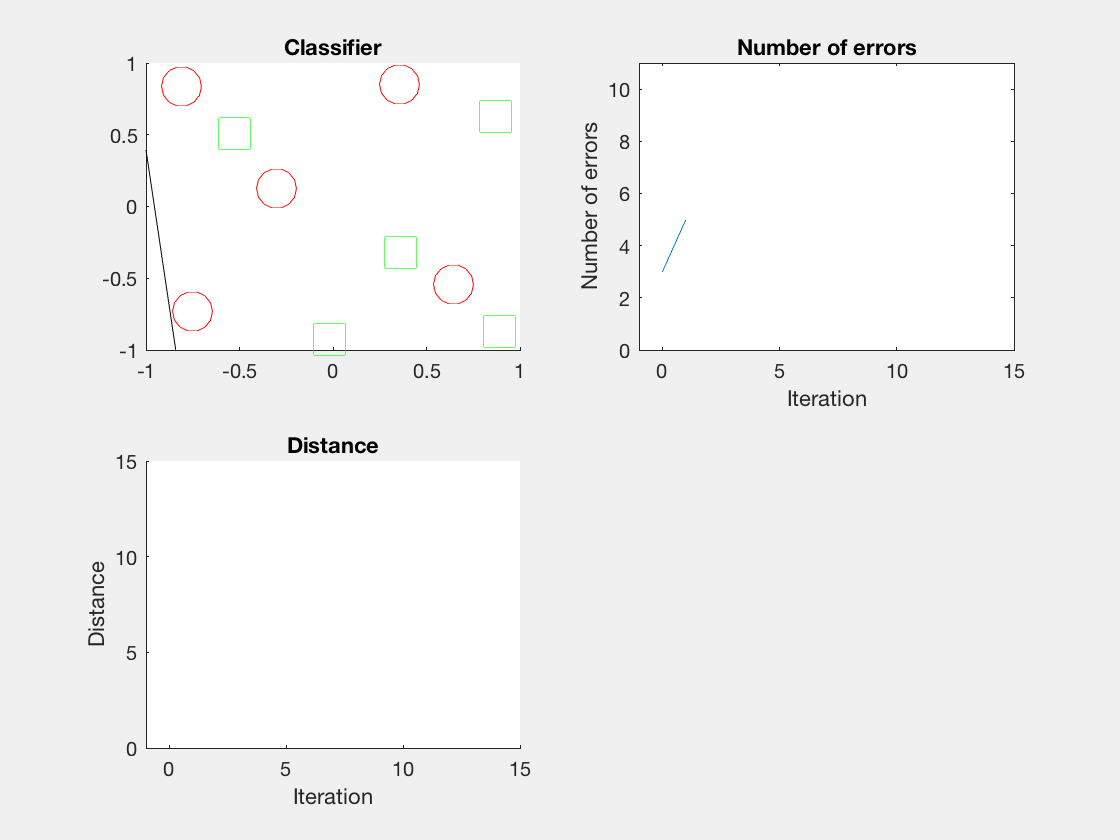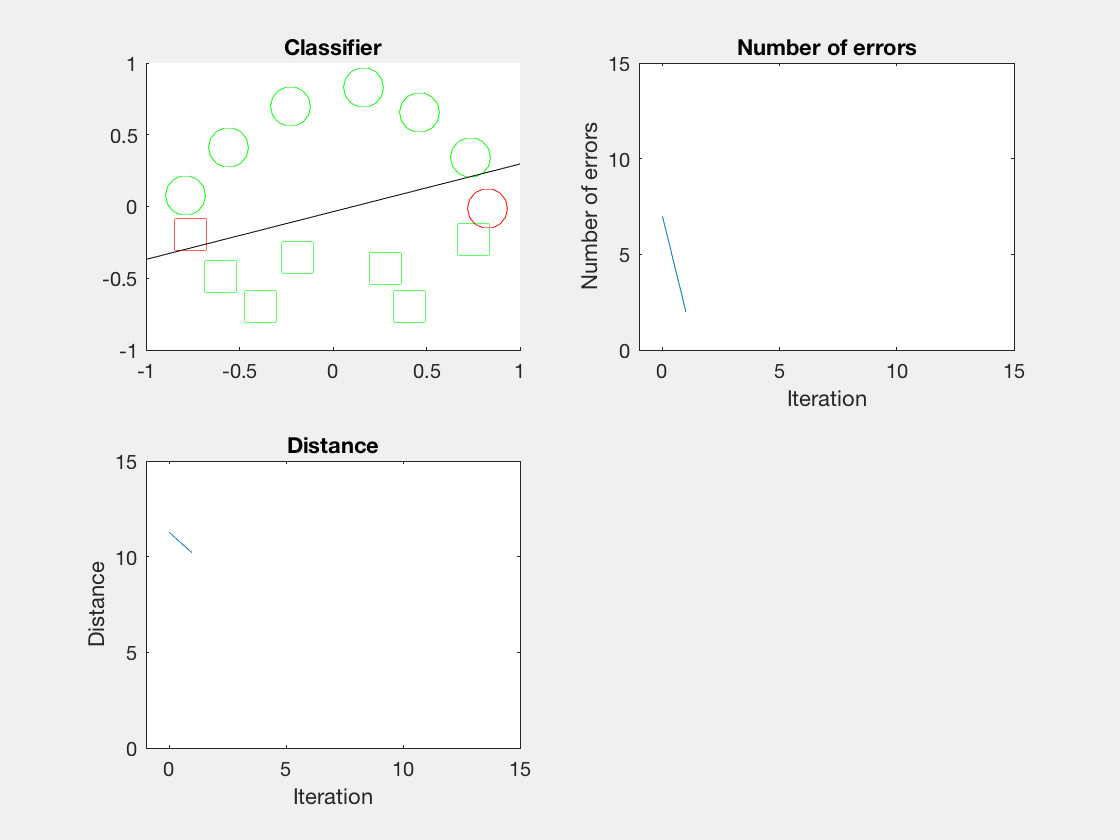## Reference

### 评论 2

1. #2

楼主威武，我正愁呢，想用python，又不是特别熟悉

curry6年前 (2017-09-24)回复
2. #1

请问楼主最后那个动图在MATLAB中怎么实现的呢？

天泽287年前 (2017-04-20)回复